A quadrilateral is a 2 – D shape which contain 4 sides. It consist of four sides, four angles and four vertices.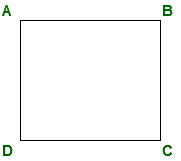Different type of quadrilateral and their properties:

1. Rectangle:
The rectangle, like the square, is one of the most commonly known quadrilaterals. It is defined as having all four interior angles 90° (right angles).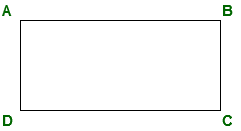• All four angles in a rectangle are 90°.
• Opposite side are parallel and of same length(or congruent).
• Diagonals of rectangle bisect each other and divide a the rectangle into two congruent triangles.
• Area of rectangle = length * breadth.
• Perimeter of rectangle = 2*(length + breadth)

2. Square:
In geometry, a square is a regular quadrilateral, which means that it has four equal sides and four equal angles (90-degree angles, or (100-gradian angles or right angles). It can also be defined as a rectangle in which two adjacent sides have equal length.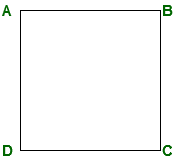• All four angles in a rectangle are 90°.
• All sides are equal and opposite sides are parallel(or congruent).
• Diagonals of square bisect each other at 90° and divide the square into two congruent triangle.
• Area of square = side * side
• Perimeter of square = 4 * side

3. Rhombus:
A Rhombus is a flat shape with 4 equal straight sides. A rhombus looks like a diamond. All sides have equal length. Opposite sides are parallel, and opposite angles are equal (it is a Parallelogram).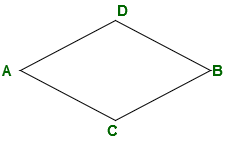• All sides are equal in a rhombus.
• Opposite angles are equal in rhombus.
• The diagonals of rhombus intersect each other at equal angles.
• Area of rhombus = (diagonal1 * diagonal2) / 2
• Perimeter of rhombus = 4 * side

4. Trapezium:
In Euclidean geometry, a convex quadrilateral with at least one pair of parallel sides is referred to as a trapezoid.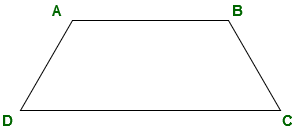• Atleast one pair of opposite side is parallel to each other.
• Area of trapezium = (sum of length of parallel side(or bases)) * height / 2.
• Perimeter of trapezium = sum of all sides.

5. Parallelogram:
In Euclidean geometry, a parallelogram is a simple quadrilateral with two pairs of parallel sides. The opposite or facing sides of a parallelogram are of equal length and the opposite angles of a parallelogram are of equal measure.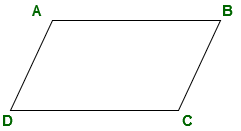• Opposite sides are parallel and congurent to each other.
• Opposite angles are congurent to each other.
• Sum of adjacent angles is 180°
• Diagonals of parallelogram bisect each other and divide the parallelogram into two congurent triangles.
• Area of parallelogram = length * breadth
• Perimeter of parallelogram = 2(length + breadth)

What will be the area and perimeter of given rectangle if length of rectangle is 5cm and breadth of rectangle is 7cm?Solution:

```Area of rectangle = length * breadth.
Area of rectangle = 5 * 7 = 35 cm2
Perimeter of rectangle = 2*(length + breadth)
Perimeter of rectangle = 2*(5 + 7) = 24cm
```

What will be the are of given rhombus if diagonal of rhombus are 4cm and 6cm?```Area of rhombus = (diagonal1 * diagonal2) / 2
Area of rhombus = (4 * 6) / 2 = 12 cm2
```

What will be area and perimeter of given trapezium if AB is parallel to CD?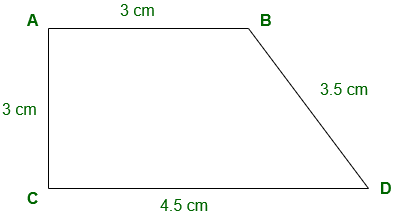```Area of trapezium = (sum of length of parallel side(or bases)) * height / 2.
Area of trapezium = (3 + 4. 5) * 3 / 2
= 11.25 cm2
Perimeter of trapezium = Sum of all sides
= 3 + 3 + 3.5 + 4.5 = 14 cm
```

My Personal Notes arrow_drop_upCheck out this Author's contributed articles.

If you like GeeksforGeeks and would like to contribute, you can also write an article using contribute.geeksforgeeks.org or mail your article to contribute@geeksforgeeks.org. See your article appearing on the GeeksforGeeks main page and help other Geeks.

Please Improve this article if you find anything incorrect by clicking on the "Improve Article" button below.

Improved By : shubham_singh

Article Tags :

Be the First to upvote.

Please write to us at contribute@geeksforgeeks.org to report any issue with the above content.